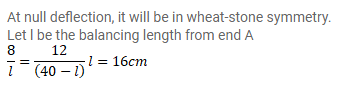# The potentiometer wire AB shown in figure (32-E26) is 40cm long.

Question:

The potentiometer wire AB shown in figure (32-E26) is $40 \mathrm{~cm}$ long. Where the free end of the galvanometer should be connected on $\mathrm{AB}$ so that the galvanometer may show zero deflection?Solution: